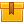# Binary Logic Circuits (A9401)

## Prerequisites

This lesson is designed for participants familiar with AC/DC theory, electrical safety, and electrical print reading. A basic understanding of logic gates is also required.

## Description

This lesson explains and demonstrates binary logic signals and the use of logic gates in integrated circuits. The lesson also describes Boolean expressions, truth tables, and Karnaugh mapping in relation to the logic of complex circuits. These topics are directly applied to troubleshooting digital circuits.

## Objectives

Identify voltages of digital components. Identify leading and trailing edges of a digital signal. Interpret pin connection diagrams and wiring diagrams. State the function of a pin on an IC chip when provided with a pin connection diagram or a wiring diagram. Properly use a logic probe, logic clip, logic monitor, and logic pulser to test the operation of an IC chip. Test the operation of a circuit and determine if it is functioning properly. Interpret and develop related Boolean expressions and truth tables. Simplify a two-variable Boolean expression using Karnaugh mapping.

## ContentThis lesson explains and demonstrates binary logic signals and the use
of logic gates in integrated circuits. The lesson also describes Boolean
expressions, truth tables, and Karnaugh mapping in relation to the
logic of complex circuits. These topics are directly applied to
troubleshooting digital circuits.

•Binary Logic Circuits

0
0 Reviews